# Civil Engineering - Applied Mechanics

### Exercise :: Applied Mechanics - Section 1

6.

A projectile is thrown at an angle a to the horizontal with α velocity v. It will have the maximum centripetal acceleration

 A. at the start B. at the top of the trajectory C. as it strikes the ground D. else where.

Explanation:

No answer description available for this question. Let us discuss.

7.

The c.g. of the shaded area of the below figure whose curve OM is a parabola from y-axis, is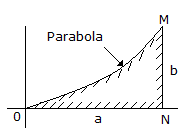A.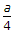B.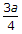C.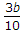D.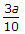E.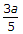Explanation:

No answer description available for this question. Let us discuss.

8.

The unit of Moment of Inertia of a body, is

 A. m B. m2 C. m3 D. m4 E. none of these.

Explanation:

No answer description available for this question. Let us discuss.

9.

If a body moves in such a way that its velocity increases by equal amount in equal intervals of time, it is said to be moving with

 A. a uniform retardation B. a uniform acceleration C. a variable acceleration D. a variable retardation E. none of these.

Explanation:

No answer description available for this question. Let us discuss.

10.

If the gravitational accelerational at any place is doubled, the weight of a body, will

 A. be reduced to half B. be doubled C. not be affected D. none of these.